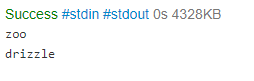In: Other

# Write a program that reads an integer, a list of words, and a character.

13.14 LAB: Contains the character

Write a program that reads an integer, a list of words, and a character. The integer signifies how many words are in the list. The output of the program is every word in the list that contains the character at least once. Assume at least one word in the list will contain the given character.

Ex: If the input is:

4 hello zoo sleep drizzle z

then the output is:

zoo
drizzle

In c++

## Solutions

##### Expert Solution

#include
#include
using namespace std;

int main() {
int n;
string str;
cin>>n;
char ch;
string arr[n];
for(int i=0;i    cin>>arr[i];
cin>>ch;
for(int i=0;i    {
str=arr[i];
for(int j=0;str[j]!='\0';j++)
{
if(str[j]==ch)
{
cout<          break;
}
}
}
return 0;
}

OUTPUT:-## Related Solutions

##### Lab 5 a) Write a program that reads in an unsigned integer K and sums the...
Lab 5 a) Write a program that reads in an unsigned integer K and sums the first K many integers that are divisible by 7. You should output the sum on a formatted manner b)Consider the following diamond it is an 11 by 11 diamond made with * signs. Write a program that takes as input positive odd integer K (greater than or equal to three and outputs a K by K diamond made with * signs * *** *...
##### Write a program that reads a file (provided as attachment to this assignment) and write the...
Write a program that reads a file (provided as attachment to this assignment) and write the file to a different file with line numbers inserted at the beginning of each line. Such as Example File Input: This is a test Example File Output 1. This is a test. (Please comment and document your code and take your time no rush).
##### Checker for integer string Forms often allow a user to enter an integer. Write a program...
Checker for integer string Forms often allow a user to enter an integer. Write a program that takes in a string representing an integer as input, and outputs yes if every character is a digit 0-9. Ex: If the input is: 1995 the output is: yes Ex: If the input is: 42,000 or 1995! the output is: no Hint: Use a loop and the Character.isDigit() function. import java.util.Scanner; public class LabProgram { public static void main(String[] args) { Scanner scnr...
##### Write a program that prompts the user to enter a positive integer and then computes the...
Write a program that prompts the user to enter a positive integer and then computes the equivalent binary number and outputs it. The program should consist of 3 files. dec2bin.c that has function dec2bin() implementation to return char array corresponding to binary number. dec2bin.h header file that has function prototype for dec2bin() function dec2binconv.c file with main function that calls dec2bin and print results. This is what i have so far. Im doing this in unix. All the files compiled...
##### Write a program that takes in a positive integer as input, and outputs a string of...
Write a program that takes in a positive integer as input, and outputs a string of 1's and 0's representing the integer in binary. For an integer x, the algorithm is: As long as x is greater than 0 Output x % 2 (remainder is either 0 or 1) x = x / 2 Note: The above algorithm outputs the 0's and 1's in reverse order. Ex: If the input is: 6 the output is: 011 6 in binary is...
##### Forms often allow a user to enter an integer. Write a program that takes in a...
Forms often allow a user to enter an integer. Write a program that takes in a string representing an integer as input, and outputs yes if every character is a digit 0-9. Ex: If the input is: 1995 the output is: yes Ex: If the input is: 42,000 or any string with a non-integer character, the output is: no PYTHON 3
##### C++ Write a program that takes a string and integer as input, and outputs a sentence...
C++ Write a program that takes a string and integer as input, and outputs a sentence using those items as below. The program repeats until the input string is "quit". If the input is: apples 5 shoes 2 quit 0 the output is: Eating 5 apples a day keeps your doctor away. Eating 2 shoes a day keeps your doctor away.
##### Write a program that takes in a positive integer as input, and outputs a string of 1's and 0's representing the integer in binary. For an integer x, the algorithm is:
USE Coral Write a program that takes in a positive integer as input, and outputs a string of 1's and 0's representing the integer in binary. For an integer x, the algorithm is:As long as x is greater than 0    Output x % 2 (remainder is either 0 or 1)    x = x / 2Note: The above algorithm outputs the 0's and 1's in reverse order.Ex: If the input is 6, the output is:011(6 in binary is 110; the algorithm outputs the bits in reverse).
##### Write a program that takes in a positive integer as input, and outputs a string of 1's and 0's representing the integer in binary. For an integer x, the algorithm is:
In Java  Write a program that takes in a positive integer as input, and outputs a string of 1's and 0's representing the integer in binary. For an integer x, the algorithm is:As long as x is greater than 0    Output x % 2 (remainder is either 0 or 1)    x = x / 2Note: The above algorithm outputs the 0's and 1's in reverse order.Ex: If the input is:6the output is:0116 in binary is 110; the algorithm outputs the bits in reverse.
##### Write in C++: create a Doubly Linked List class that holds a struct with an integer...
Write in C++: create a Doubly Linked List class that holds a struct with an integer and a string. It must have append, insert, remove, find, and clear.